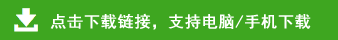# 小学二年级数学上册期末测试题及答案

小学二年级数学上册期末测试题及答案部分内容预览

一、基础知识。
1、填上合适的单位：
（1）小刚手指的宽大约是1（   ）     （2）大树高约4（   ）
（3）一支毛笔的长大约是20（   ）    （4）笑笑的身高是100（    ）
2、看图填空。
乘法算式是：（                   ） ，表示（  ）个（  ）相加，又表示（  ）个（  ）相加，口诀是（            ）。
3、比45多5的数是（     ），比38少6的数是（     ）。
4、看线段图填空
芳芳给明明5个皮球后，两人就一样多，原来两人差（    ）个皮球。
5、在○里填上“＞”、“＜”或“﹦”。
55+4○6×9        3×9○50-22           100厘米○1米
6、下面是二、一班同学最喜欢吃的水果调查统计图。请根据统计图回答问题：
（1） 喜欢吃（    ）的人最少。
（2）喜欢吃西瓜和苹果的一共（    ）人。
（3）本次一共调查了（   ）人。
二、计算天地。
1.直接写得数。18分
8×6=       4+20=      78-20=      1×1=        67+0=
35+40=    92-0=     4×9=        50-25=       77-70=
29+3=      6×7=      7×1=        9+9=        4×6=
2×6+20=              7×8-16=                 8×4+8=
2.笔算。14分
27+48=       97-39=      34+26-18=
45-37=       34+27=      18+27+55=
三、操作乐园。
1、仔细观察，在正确位置连线。
2、上面哪个茶壶是下面那个茶壶，在镜子里看到的图像，打个对号。
3、画出下面图形的对称轴。
4、下图中共有（   ）个角，在图中画一条线段，使它增加两个直角。
5.按要求涂色。圆圈涂3个，方框的个数是圆圈的2倍，三角的个数是5倍。
6.我会分辨（对的打“√”错的打“×”）
（1）一个角的两条边开口越大，这个角就越大。                （   ）
（2）小明的身高是135厘米。                                （   ）
（3）3×4+4可以看作4×4=16                                （   ）
（4）厘米尺上从刻度4到刻度9之间长5厘米。                 （   ）
（5） 2连续加，得出18，需要加9次。                              （   ）
（6）杨树有8棵，柳树的棵树是杨树的6倍，柳树有几棵？列式是 48÷8=6棵（     ）